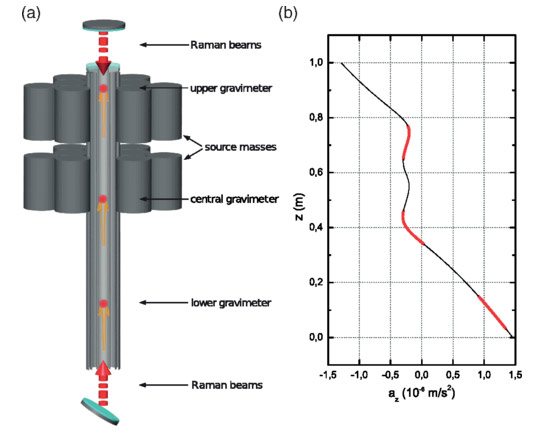# Physicists Use Atom Interferometry to Directly Measure Gravity’s Curvature(a) Scheme of the experiment. Rb87 atoms are trapped and cooled in a MOT. Three atomic clouds are launched in rapid sequence along the vertical direction with a moving optical molasses. Near the apogees of the atomic trajectories, a measurement of the vertical acceleration sensed by the three clouds is performed by Raman interferometry. External source masses are positioned in order to maximize the average gravity curvature at the three clouds’ positions. (b) Gravitational acceleration along the symmetry axis (az) produced by the source masses and the Earth’s gravity gradient; a constant value accounting for the Earth’s gravitational acceleration was subtracted. The spatial regions of the three atom interferometers are indicated by the thick red lines. Credit: American Physical Society, doi:10.1103/PhysRevLett.114.013001

A newly published study details how a team of physicists simultaneously measured gravity with cold atoms at three different heights to achieve the first direct measurement of the gravity-field curvature.

Earth’s gravitational pull gradually decreases with increasing altitude, and researchers have detected the differences even over several vertical feet within a lab, using the extreme sensitivity of cold atoms. Now a team has taken the next step by measuring the change in this gravity gradient produced by a large mass, using measurements at three different heights. They say their technique could improve gravity-based mapping of variations in rock density in geology and prospecting, and it could also boost the precision of tests of general relativity and measurements of the gravitational constant.

The technique of atom interferometry enables distance measurements with extremely high precision, by exploiting the atoms’ quantum-mechanical wavelike nature. It has been used previously to measure the strength of gravitational fields and also the rate of change in those fields over some distance (the gradient). Together such measurements permit Newton’s gravitational constant G to be determined [1, 2]. It is currently known to within about 100 parts per million, a much lower precision than other fundamental constants. More accurate measurements would allow higher-precision tests of the theory of general relativity.

Measuring gravity at two close locations gives the gradient as the difference between the two divided by their separation distance; measuring at three locations gives the rate of change of the gradient, which is also called the curvature of the field. This experiment was proposed in 2002 , and now a team in Italy, led by Guglielmo Tino of the University of Florence and the National Institute of Nuclear Physics (INFN), has carried it out. Previously, Tino and his colleagues determined G by measuring gravity at two different heights with a similar experiment .

To measure gravity at three locations simultaneously, the team launched three clouds of ultracold atoms to three different heights inside a meter-long vertical pipe. Surrounding the top half of the pipe was 516kg (1,138lb) of tungsten alloy weights, to increase the variation in the gravitational field. Near the peaks of their trajectories, the atoms were irradiated with a rapid series of laser pulses from the top and bottom of the pipe.

In the team’s technique, the first pulse separates each cloud into two populations—one that absorbs two photons, sending it into an excited state and also providing a momentum boost, and a second population that remains in the ground state. The extra momentum causes the first population to fall a different distance during a fixed time, which leads to a gravity-dependent difference in the number of quantum wave cycles that elapse, compared with the ground-state population. Two more wave pulses recombine the populations, allowing them to interfere. From the interference effects the researchers can calculate the difference in the lengths of the two populations’ trajectories, a difference that depends on the gravitational acceleration. The team measured variations in the gravitational acceleration of a few millionths of a percent and calculated the average curvature to be 1.4×10−5s−2m−1, which is virtually identical to the value they predicted.

Measuring the curvature of a gravitational field could improve the measurement of G, says Tino. A common method involves measuring the field strength and gradient as a heavy mass is moved between one detector and another. But by making two separate measurements of the gradient at different positions simultaneously, the new technique could eliminate systematic sources of error without having to move the mass, which can introduce errors from shifts of the apparatus.

The curvature could also be useful for mapping gravity changes in the earth, which are used to deduce buried geological structures and to find oil reservoirs. Even if the density changes are small, the curvature can alter dramatically if the density change is abrupt, like a step edge. So measuring gravity curvature could improve the spatial resolution of such density maps.

“Measuring the gravitational force is sensitive to everything underground,” says Holger Müller of the University of California at Berkeley, who uses atom interferometry to make ultraprecise measurements for probing fundamental physics. “Measuring the gravity gradient enhances the sensitivity to nearby objects, and measuring the [curvature] does so even more.” A practical, curvature-measuring device would be “a great achievement,” Müller says.

Reference: “Measurement of the Gravity-Field Curvature by Atom Interferometry” by G. Rosi, L. Cacciapuoti, F. Sorrentino, M. Menchetti, M. Prevedelli and G. M. Tino, 5 January 2015, Physical Review Letters.
DOI: 10.1103/PhysRevLett.114.013001

Notes:

1. “Atom Interferometer Measurement of the Newtonian Constant of Gravity” by J. B. Fixler, G. T. Foster, J. M. McGuirk and M. A. Kasevich, 5 January 2007, Science.
DOI: 10.1126/science.1135459
2. “Determination of the Newtonian Gravitational Constant Using Atom Interferometry” by G. Lamporesi, A. Bertoldi, L. Cacciapuoti, M. Prevedelli and G. M. Tino, 8 February 2008, Physical Review Letters.
DOI: 10.1103/PhysRevLett.100.050801
3. “Sensitive absolute-gravity gradiometry using atom interferometry” by J. M. McGuirk, G. T. Foster, J. B. Fixler, M. J. Snadden and M. A. Kasevich, 8 February 2002, Physical Review A.
DOI: 10.1103/PhysRevA.65.033608
4. “Precision measurement of the Newtonian gravitational constant using cold atoms” by G. Rosi, F. Sorrentino, L. Cacciapuoti, M. Prevedelli and G. M. Tino, 18 June 2014, Nature.
DOI: 10.1038/nature13433